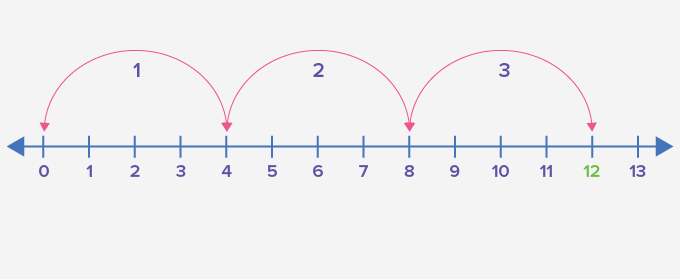Multiplier - Definition with Examples

The Complete K-5 Math Learning Program Built for Your Child

• 30 Million Kids

Loved by kids and parent worldwide

• 50,000 Schools

Trusted by teachers across schools

• Comprehensive Curriculum

Aligned to Common Core

What is Multiplier?

Multiplication is one of the four basic operations of arithmetic. It gives the result of combining groups of equal sizes.Here each group has 6 candies in it, and there are 3 such groups. So, there are 3 times 6 or 6 + 6 + 6 or 18 candies in total.

Multiplication of two numbers gives ‘product’. The number of objects in each group is called ‘multiplicand,’ and the number of such equal groups is called ‘multiplier’. It is represented by cross ‘×’, asterisk ‘*’ or dot ‘·’.

For example:In the horizontal way of writing a multiplication statement, the multiplier is the leftmost number.In the vertical column method of multiplication, the multiplier is the number on top.The meaning of the word multiplier is a factor that amplifies or increases the base value of something else.  For example, in the multiplication statement 3 × 4 = 12 the multiplier 3 amplifies the value of 4 to 12.

But it need not be the case always.

If the multiplier is one, the value of the multiplicand remains the same in the product. If the multiplier is less than 1, for example, if the multiplier is 12, it reduces the value of multiplicand in the product.

12×10=5

If the multiplier is zero, the value of the multiplicand gets reduced to zero in product.

When we represent multiplication on a number line, the multiplier is the number of jumps that it takes to reach the product.

For example:

3 × 4 = 12Fun Facts: When we multiply two numbers the order does not matter. That is, 2 × 3 = 3 × 2. 2 × 3 = 3 + 3 = 6 3 × 2 = 2 + 2 + 2 = 6

Thus, when we change the order in which the numbers are multiplied, the multiplier and multiplicand get interchanged.

Won Numerous Awards & Honors